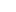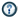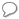xNumerical analysisOverview

Numerical analysis is the study of algorithm
Algorithm
In mathematics and computer science, an algorithm is an effective method expressed as a finite list of well-defined instructions for calculating a function. Algorithms are used for calculation, data processing, and automated reasoning...

s that use numerical approximation
Approximation
An approximation is a representation of something that is not exact, but still close enough to be useful. Although approximation is most often applied to numbers, it is also frequently applied to such things as mathematical functions, shapes, and physical laws.Approximations may be used because...

(as opposed to general symbolic manipulations
Symbolic computation
Symbolic computation or algebraic computation, relates to the use of machines, such as computers, to manipulate mathematical equations and expressions in symbolic form, as opposed to manipulating the approximations of specific numerical quantities represented by those symbols...

) for the problems of mathematical analysis
Mathematical analysis
Mathematical analysis, which mathematicians refer to simply as analysis, has its beginnings in the rigorous formulation of infinitesimal calculus. It is a branch of pure mathematics that includes the theories of differentiation, integration and measure, limits, infinite series, and analytic functions...

(as distinguished from discrete mathematics
Discrete mathematics
Discrete mathematics is the study of mathematical structures that are fundamentally discrete rather than continuous. In contrast to real numbers that have the property of varying "smoothly", the objects studied in discrete mathematics – such as integers, graphs, and statements in logic – do not...

).

One of the earliest mathematical writings is the Babylonian tablet BC 7289, which gives a sexagesimal numerical approximation of, the length of the diagonal in a unit square.